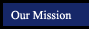Algebra 1 - Prentice-Hall Algebra 1 California EditionChapter 1 Introduction to Algebra Chapter 2 Integers and Rational Numbers Chapter 3 Equations Chapter 4 Inequalities Chapter 5 Exponents and Polynomials Chapter 6 Polynomials and Factoring Chapter 7 Graphs and Linear Equations Chapter 8 Systems of Equations Chapter 9 Inequalities and Absolute Value Chapter 10 Rational Expressions and Equations Chapter 11 Radicals Expressions and Equations Chapter 12 Relations and Functions Chapter 13 Quadratic Equations